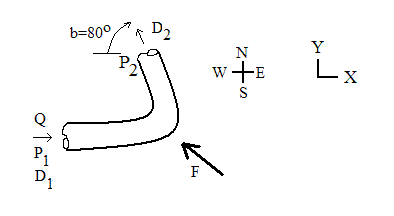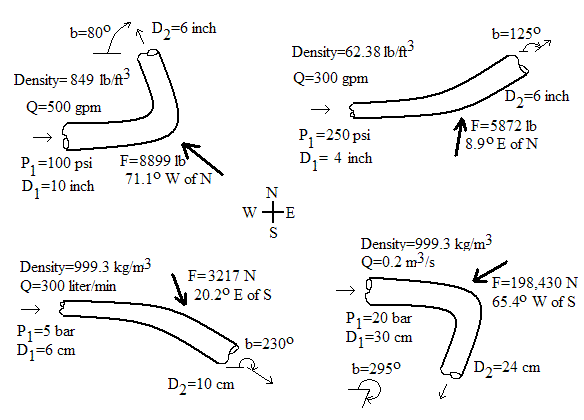Force required to hold a pipe bend in place

Compute reaction force. Thrust block sizing. Incompressible fluid flowing through a horizontal pipe bend.

Register to enable "Calculate" button. Demonstration mode for mercury

 Select fluid and units: Fluid density (lb/ft3): Water at 68F, 20C Water at 55F, 13C Water at 39F, 4C Seawater at 60F, 16C SAE 30 oil at 60F, 16C Mercury at 68F, 20C User enters density Flowrate, Q (gpm): Density in kg/m3 Density in g/cm3 Density in N/m3 Density in slug/ft3 Density in lb/ft3 Pipe angle, b (degree): Flowrate in m3/s Flowrate in m3/min Flowrate in liter/s Flowrate in liter/min Flowrate in ft3/s Flowrate in ft3/min Flowrate in gal/min (US) Flowrate in gal/hr (US) Flowrate in gal/day (US) Flowrate in MGD Upstream diameter, D1 (inch): Angle in radian Angle in degree Downstream diameter, D2 (inch): Diameter in cm Diameter in meter Diameter in inch Diameter in foot Upstream pressure, P1 (psi): Pressure in millibar Pressure in bar Pressure in N/m2 or Pa Pressure in mm Mercury (0C) Pressure in psi Pressure in inch H2O (60F) Pressure in inch Mercury (60F) Downstream pressure, P2 (psi): Force in dyne Force in Newton Force in pound Force magnitude, F (lb): Velocity in m/s Velocity in ft/s Force direction: x-component of Force, Fx (lb): © 2014 LMNO Engineering, y-component of Force, Fy (lb): Research, and Software, Ltd. Upstream velocity, V1 (ft/s): http://www.LMNOeng.com Downstream velocity, V2 (ft/s):

Units for force on pipe bend calculation: cfm=cubic feet per minute, cfs=cubic feet per second, cm=centimeter, g=gram, ft=foot, gal=gallon (US), gpd=gallons (US) per day, gph=gallons (US) per hour, gpm=gallons (US) per minute, Hg=mercury, hr=hour, H2O=water, in=inch, kg=kilogram, lb=pound, m=meter, mbar=millibar, MGD=Million gallons (US)/day, min=minute, mm=millimeter, N=Newton, Pa=Pascal, psi=pound per square inch, s=second.Discussion
In order to properly size thrust blocks or other devices to hold a pipe in place, the momentum equation is used to compute the necessary resistive force to hold the pipe stationary. This is also called thrust restraint design. Forces in a pipe bend in the horizontal plane are caused by the fluid's momentum and pressure. If the pipe undergoes a bend in the vertical plane, where the entrance to the bend is above the exit (or vice-versa), then the weight of the liquid and pipe material within the bend will contribute to the force. Since computing the volume of fluid and pipe material within a bend requires considerably more input, we kept our calculation relatively simple by keeping it in the horizontal plane. Our calculation is also valid for incompressible gases, but - due to a gas's low density - the force required to hold a gas pipe in place is typically small compared to the force required to hold a liquid pipe in place unless pressures are high. The forces Fx and Fy computed by the calculation are the x and y components of the total force F.

The four diagrams below further explain the bend angle b and the direction of the computed force for various situations. The pipe bends are in the horizontal plane. Plain arrow (-->) indicates flow direction. Bold arrow (-->) indicates resistive force that must be applied to keep the bend in place.Equations for force on pipe bend calculation
The equations used in our calculation can be found in nearly any college level fluid mechanics textbook (e.g. Munson et al., 1998) or fluid mechanics reference handbook. The force equations are based on linear momentum conservation. P2 is computed using the Bernoulli Equation, which assumes negligible friction loss around the bend.

Subscript 1 is upstream of bend; Subscript 2 is downstream of bend; Subscript x is x-component of force; Subscript y is y-component of force. For a pipe bend in the horizontal plane where friction effects around the bend are negligible:

Fx = -P1A1 - P2A2 cos(b) - d Q [V1 + V2 cos(b)]

Fy = P2 A2 sin(b) + d V2 Q sin(b)     F = (Fx2 + Fy2)1/2

Q=VA       A=π D2 / 4

P2 = P1 + d (V12 - V22) / 2

Variables
To use the equations above, a consistent set of units must be used. The variables below show the SI (System International) units for each variable. Our calculation allows a variety of other units with the conversions made internally within the program.
A=Pipe flow area (m2)
b=Pipe angle shown in the figure above (valid range is 0 to 2π radians or 0 to 360o)
d=Liquid (or gas) mass density (kg/m3)
D=Pipe diameter (m)
F=Resistive (reaction) force (N)
P=Gage pressure (N/m2, relative to atmospheric pressure)
Note: P1 and P2 cannot physically be below 0.0 absolute pressure (-101,325 N/m2 gage). Further, if P1 or P2 approach the liquid's vapor pressure, flashing to vapor may occur and the calculation will not be accurate. Vapor pressure is not checked by the program, but a message will appear if P1 or P2 is less than -101,325 N/m2 gage.
Q=Flowrate (m3/s)
V=Velocity (m/s)
π=Greek letter pi, 3.1415926....

Messages given by calculation
"Need Density>0", "Need Q>0", "Need D1>0", "Need D2>0", "Need pipe angle b>0", "Need P1>0 absolute", "Need 0 < b < 6.28 radian", "Need 0 < b < 360 degrees". These are initial checks of input data.

"Need P2>0 absolute". Message will appear after computing downstream pressure. Physically, pressure cannot be less than 0.0 absolute, which is a complete vacuum. The calculation method does not account for liquid flashing to vapor if the pressure drops below the liquid's vapor pressure.

Reference
Munson, Bruce R., Donald F. Young, and Theodore H. Okiishi. 1998. Fundamentals of Fluid Mechanics. John Wiley and Sons, Inc. 3ed.